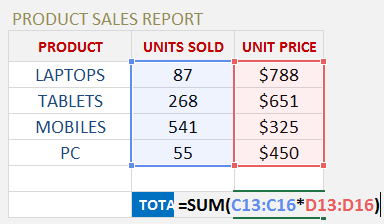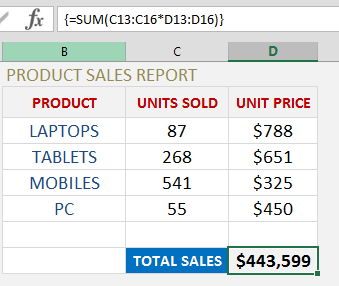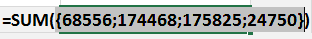An Array Formula performs an Excel operation (math, comparative, join, or function argument) on an array or range of data.  This could be a range of cells, a worksheet reference, or a defined name.

#### One of the most popular uses of an array formula is to calculate Total Sales in Excel.

If we had to calculate Total Sales in Excel the normal way, we would have to create a “helper column” for the Totals column and then enter a formula to Sum all the Totals.

Using an Array Formula we skip the “helper column” and just enter one formula only.  Quick and simple!Watch the YouTube tutorial to learn How to Calculate Total Sales Formula:Let’s do an example of an Array Formula to Calculate Total Sales in Excel for various products.

Follow the step-by-step guide below on How to calculate Total Sales in Excel:

STEP 1: Enter the SUM formula =SUM(

STEP 2: Select the array/range of data for the UNITS SOLD, enter the multiplier sign * and select the array/range of the data for the UNIT PRICE:

=SUM(C13:C16*D13:D16)STEP 3: Instead of pressing ENTER to evaluate the formula, you need to press CTRL+SHIFT+ENTER to turn the formula into an Array Formula which will look like this:

{=SUM(C13:C16*D13:D16)}STEP 4: By pressing F9 on the selected formula array will give you the resulting array of numbers (press CTRL+Z to get out of this mode when you are done checking the formula results):If we had to get the above result using a non-Array Formula we would have to create a helper column which multiplies UNITS SOLD by UNIT PRICE and then enter the SUM formula to get the same result.  This is double the work!